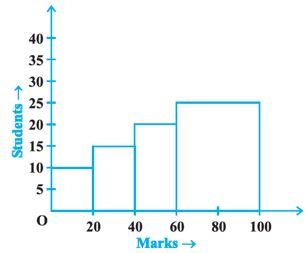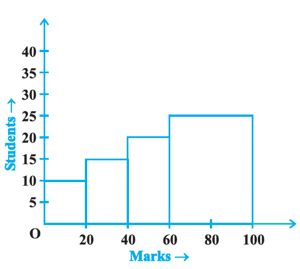Filter By

## All Questions

#### As the number of tosses of a coin increase, the ratio of the number of heads to the total number of tosses will be 0.5. Is it correct? If not, write the correct one.

As the number of tosses of a coin increase, we may have a head or a tail as an outcome. Probability of 0.5 is achieved when the total number of heads = total number of tails. So obviously we can say that it is not necessary that the favourable outcomes of tails will be equal to favourable outcomes of heads.

So this ratio may or may not be 0.5.

So we are not sure what the ratio will be.

Hence the given statement is not true.

#### Can the experimental probability of an event be greater than 1? Justify your answer.

We know that,

As we know the value of favourable outcomes can never be greater than the total outcomes.

So the probability can never be greater than 1. The probability of an event can be between only 0 and 1.

Hence, the experimental probability of an event can never be greater than 1

## Crack CUET with india's "Best Teachers"

• HD Video Lectures
• Unlimited Mock Tests
• Faculty Support#### Can the experimental probability of an event be a negative number? If not, why?

Probability of a number is determined by:  Favourable Events / Total Events

Now, the number of trials in which the event can happen cannot be negative. So the total number of trials is always positive. Hence, the experimental probability of an event is always positive.

#### 30 children were asked about the number of hours they watched TV programs last week. The results are recorded as under : Number of hours 0-5 5-10 10-15 15-20 Frequency 8 16 4 2 Can we say that the number of children who watched TV for 10 or more hours a week is 22 ? Justify your answer.

Total number of students, who watched TV for 10 or more hours are:

From the table:

C.I. = frequency

10-15= children

10-20 children

Total = 6 children

Hence, the total number of students who watched TV for 10 or more hours are 6 and not 22.

## Crack NEET with "AI Coach"

• HD Video Lectures
• Unlimited Mock Tests
• Faculty Support#### The class marks of a continuous distribution are :Is it correct to say that the last interval will be 1.55 - 1.73? Justify your answer.

Answer: As we know that class is the mid-point of a class interval.

So, Difference between two consecutive class marks is always equal to the class size.

 Class-marks Difference 1.14-1.04 0.1 1.24-1.14 0.1 1.34-1.24 0.1 1.44-1.34 0.1 1.54-1.44 0.1 1.55-1.73 0.8

So, we can see that the last class size is 0.8, which is not equal to the class size found previously.

Hence it cannot be the correct class interval for the given class marks.

#### Is it correct to say that in a histogram, the area of each rectangle is proportional to the class size of the corresponding class interval? If not, correct the statement.

In a histogram, the area of each rectangle is proportional to the frequency of its class.

The frequency of a class may change for any class interval. But for a specific class, the class size will remain the same.

We know that the frequency is represented on the y-axis and class size on the x-axis.

So we can say that for all the rectangles of a histogram, width (class size) remains the same & length (frequency) changes.

Hence, the correct statement is that in a histogram, the area of each rectangle is proportional to the frequency of its class.

## Crack JEE Main with "AI Coach"

• HD Video Lectures
• Unlimited Mock Tests
• Faculty Support#### A football player scored the following number of goals in the 10 matches: 1, 3, 2, 5, 8, 6, 1, 4, 7, 9Since the number of matches is 10 (an even number), therefore, the medianIs it the correct answer and why?

Answer : [No, as the data is not arranged in ascending order before finding the middle terms]

Given terms : 1, 3, 2, 5, 8, 6, 1, 4, 7, 9

To calculate the median, arrange the given data in ascending order and then find the middle term. This middle term is called the median.

Firstly, we will arrange the data in ascending order as:

Here the total number of observations, n=10 which is even.

In case of even number of terms:

Hence, the median is 4.5

#### A child says that the median of 3, 14, 18, 20, 5 is 18. What doesn’t the child understand about finding the median?

Answer: The child has not arranged the data in ascending order before finding the median

To calculate the median, arrange the given data in ascending order and then find the middle term. This middle term is called the median.

Firstly, we will arrange the given data in ascending order as,

Total number of terms = 5( odd )

(where n is the number of terms)

So the median is not 18

The child has not arranged the data in ascending order before finding the median.

## Crack CUET with india's "Best Teachers"

• HD Video Lectures
• Unlimited Mock Tests
• Faculty Support#### In a diagnostic test in mathematics given to students, the following marks (out of 100) are recorded:Which ‘average’ will be a good representative of the above data and why?

Given data is :

The mode is best used with categorical data.

Mean is best used with continuous and symmetrical data.

Median is best used with skewed distributions as we can find the middle of the data set.

In ascending order, we have:

In this case,

Median will be a good representative of data, because each value occurs once, hence we can draw a line right at the middle of the data.

Hence the correct answer is median.

#### The frequency distribution : Marks 0-20 20-40 40-60 60-100 Number of Students 10 15 20 25 has been represented graphically as follows :Do you think this representation is correct? Why?

Given :

 Marks 0-20 20-40 40-60 60-100 Number of Students 10 15 20 25

 Class interval (x-axis) Frequency (y-axis) 0-20 10 20-40 15 40-60 20 60-100 25

The above will be plotted as:Hence, the given graph is correct.